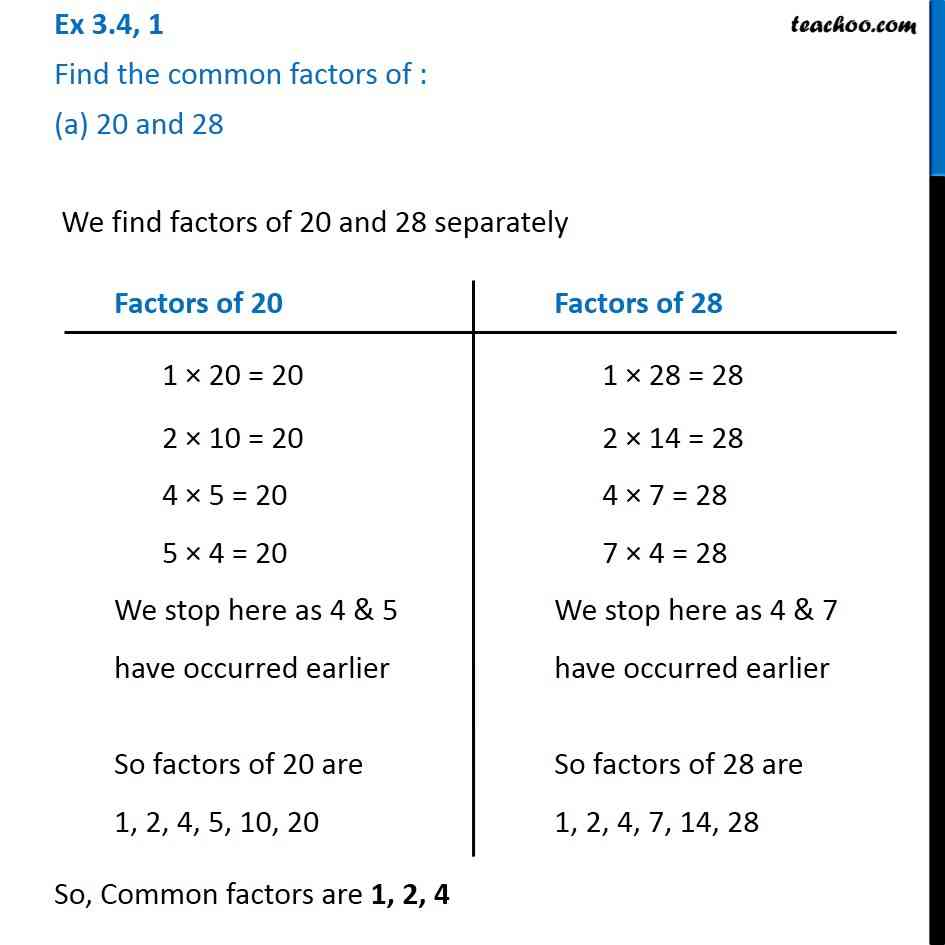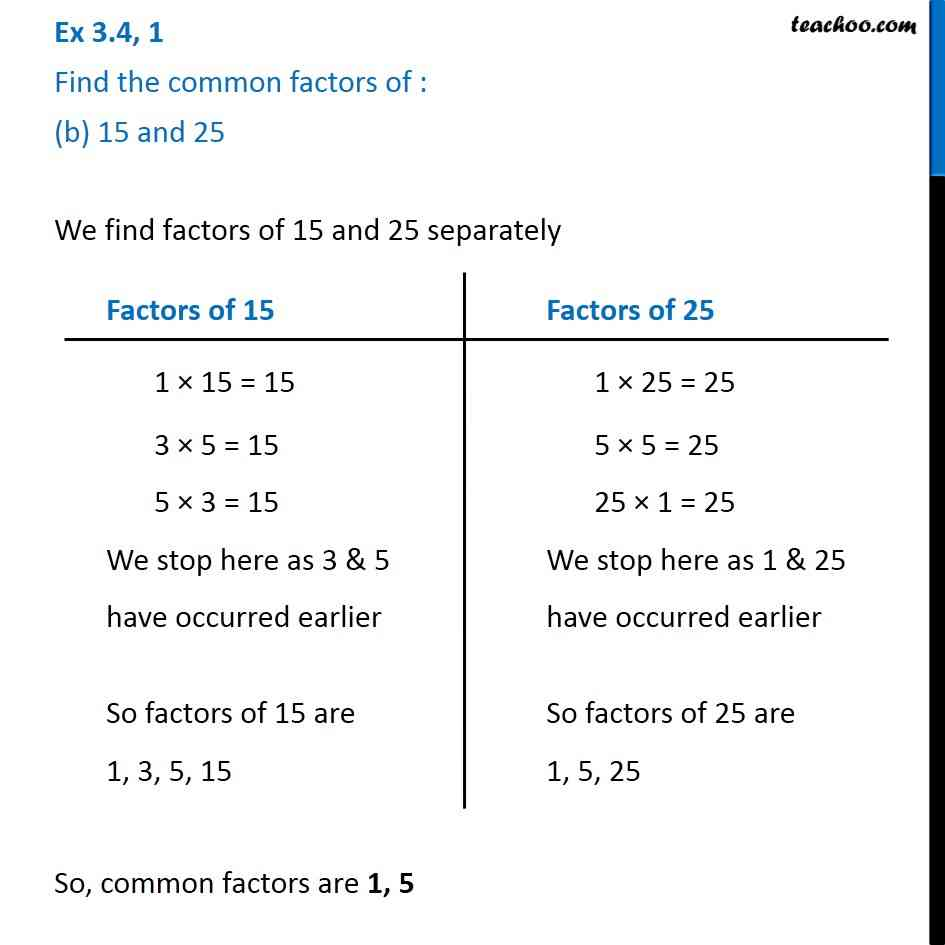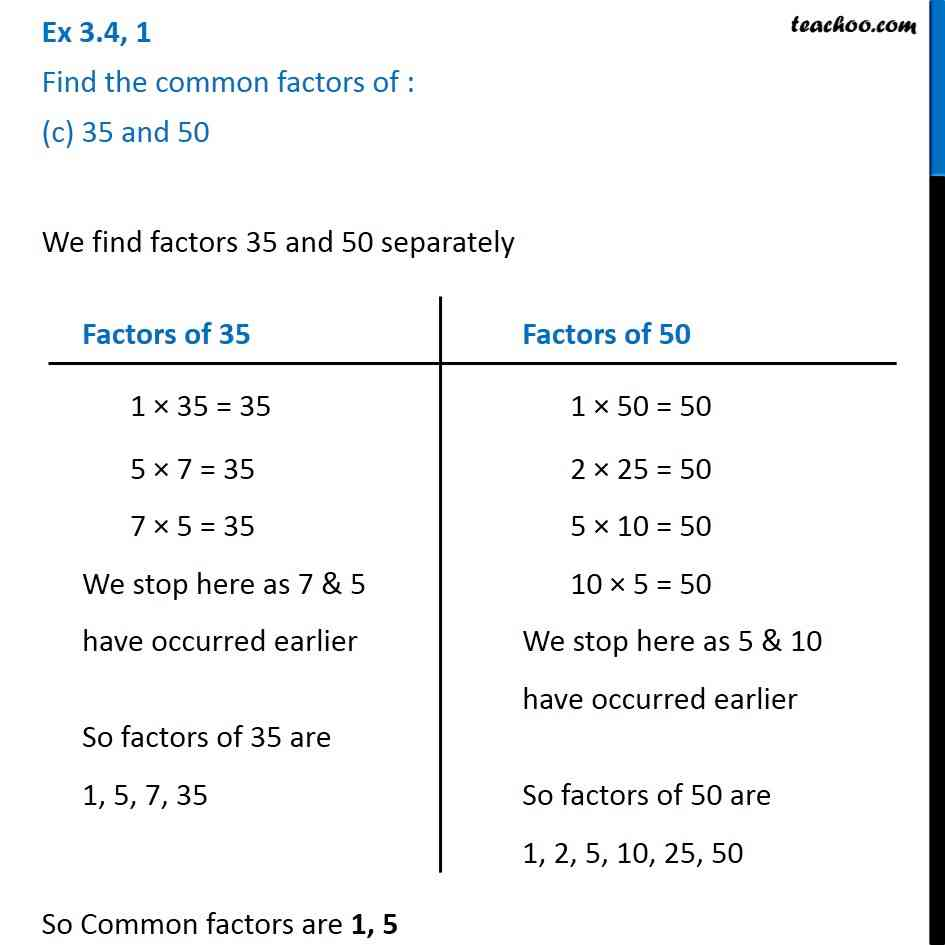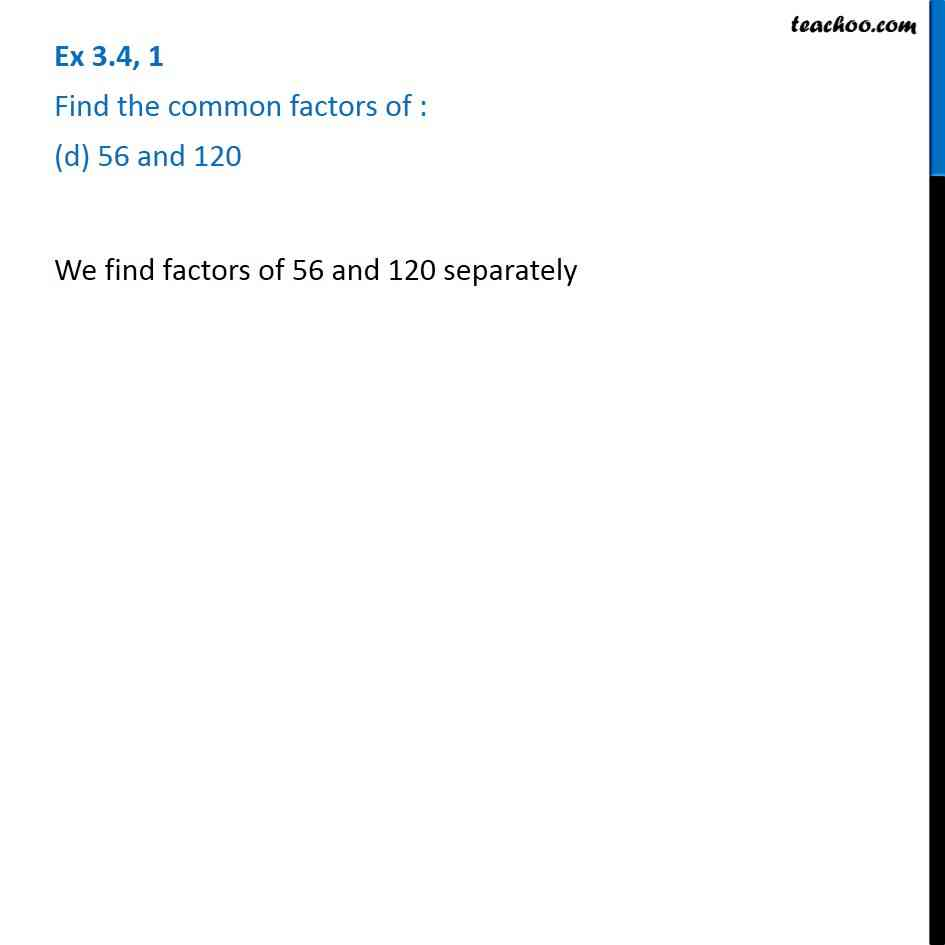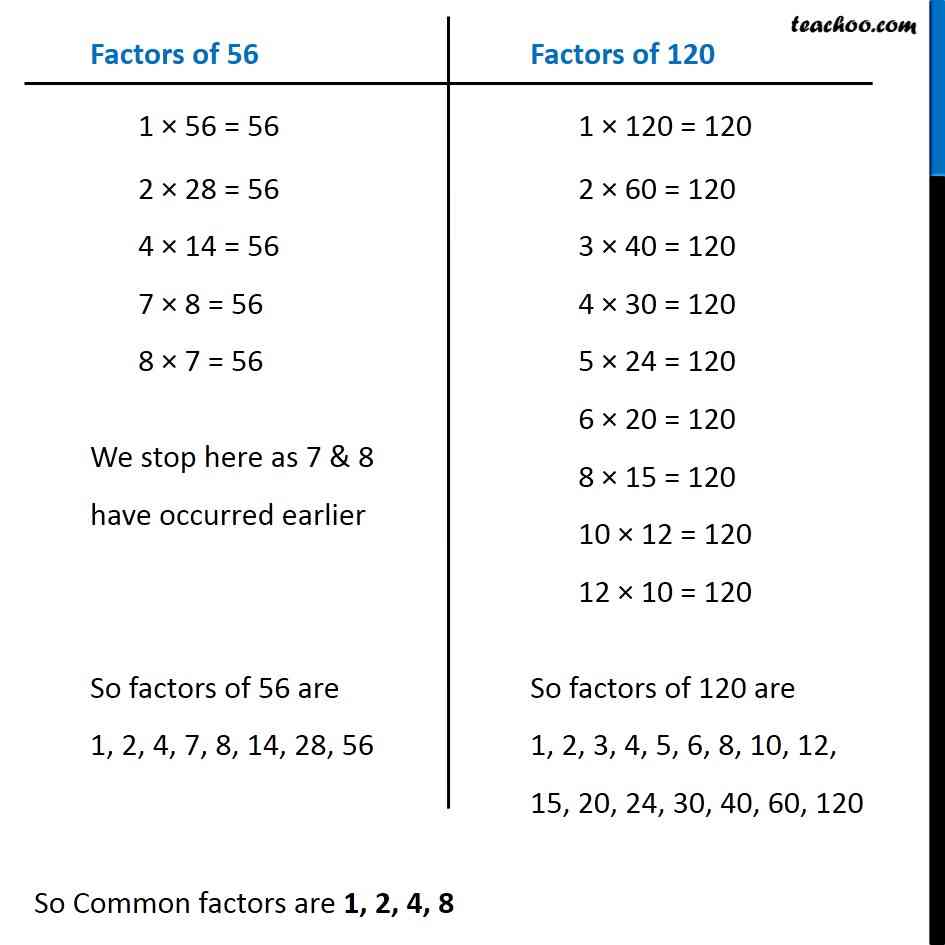1. Chapter 3 Class 6 Playing with Numbers
2. Concept wise
3. Common Factors

Transcript

Ex 3.4, 1 Find the common factors of : (a) 20 and 28 We find factors of 20 and 28 separately So, Common factors are 1, 2, 4 Factors of 20 1 × 20 = 20 2 × 10 = 20 4 × 5 = 20 5 × 4 = 20 We stop here as 4 & 5 have occurred earlier So factors of 20 are 1, 2, 4, 5, 10, 20 Factors of 28 1 × 28 = 28 2 × 14 = 28 4 × 7 = 28 7 × 4 = 28 We stop here as 4 & 7 have occurred earlier So factors of 28 are 1, 2, 4, 7, 14, 28 Factors of 28 1 × 28 = 28 2 × 14 = 28 4 × 7 = 28 7 × 4 = 28 We stop here as 4 & 7 have occurred earlier So factors of 28 are 1, 2, 4, 7, 14, 28 Factors of 28 1 × 28 = 28 2 × 14 = 28 4 × 7 = 28 7 × 4 = 28 We stop here as 4 & 7 have occurred earlier So factors of 28 are 1, 2, 4, 7, 14, 28 Factors of 28 1 × 28 = 28 2 × 14 = 28 4 × 7 = 28 7 × 4 = 28 We stop here as 4 & 7 have occurred earlier So factors of 28 are 1, 2, 4, 7, 14, 28 Ex 3.4, 1 Find the common factors of : (b) 15 and 25 We find factors of 15 and 25 separately So, common factors are 1, 5 Factors of 15 1 × 15 = 15 3 × 5 = 15 5 × 3 = 15 We stop here as 3 & 5 have occurred earlier So factors of 15 are 1, 3, 5, 15 Factors of 25 1 × 25 = 25 5 × 5 = 25 25 × 1 = 25 We stop here as 1 & 25 have occurred earlier So factors of 25 are 1, 5, 25 Ex 3.4, 1 Find the common factors of : (c) 35 and 50 We find factors 35 and 50 separately So Common factors are 1, 5 Factors of 35 1 × 35 = 35 5 × 7 = 35 7 × 5 = 35 We stop here as 7 & 5 have occurred earlier So factors of 35 are 1, 5, 7, 35 Factors of 50 1 × 50 = 50 2 × 25 = 50 5 × 10 = 50 10 × 5 = 50 We stop here as 5 & 10 have occurred earlier So factors of 50 are 1, 2, 5, 10, 25, 50 Ex 3.4, 1 Find the common factors of : (d) 56 and 120 We find factors of 56 and 120 separately Factors of 56 1 × 56 = 56 2 × 28 = 56 4 × 14 = 56 7 × 8 = 56 8 × 7 = 56 We stop here as 7 & 8 have occurred earlier So factors of 56 are 1, 2, 4, 7, 8, 14, 28, 56 Factors of 120 1 × 120 = 120 2 × 60 = 120 3 × 40 = 120 4 × 30 = 120 5 × 24 = 120 6 × 20 = 120 8 × 15 = 120 10 × 12 = 120 12 × 10 = 120 So factors of 120 are 1, 2, 3, 4, 5, 6, 8, 10, 12, 15, 20, 24, 30, 40, 60, 120

Common Factors

About the AuthorDavneet Singh
Davneet Singh is a graduate from Indian Institute of Technology, Kanpur. He has been teaching from the past 10 years. He provides courses for Maths and Science at Teachoo.